# The BioMassters Challenge Starter Code

Joining us today is Grace Woolson, who joined the student programs team in June 2022 to support data science challenges and help participants use MATLAB to win! Grace will talk about a new data science challenge that launches today November 1, 2022 with our partner DrivenData. Grace, over to you..

## Getting Started with MATLAB

Hello all, my name is Grace Woolson and today we will be talking about a new Data Science Challenge for you to test your skills! We at MathWorks®, in collaboration with DrivenData, are excited to bring you this challenge. The objective of this challenge is to estimate the annual aboveground biomass (AGBM) in a given patch of Finland when provided satellite imagery of that patch. In this blog we are providing a basic starter example in MATLAB®. In this code, I create a basic image-to-image regression model and train it to predict peak AGBM for each pixel in the input data. Then I use this model on test data and save the results in the format required for the challenge.
This should serve as basic starting code to help you to start analyzing the data and work towards developing a more efficient, optimized, and accurate model using more of the training data available. To request your complimentary MATLAB license and other getting started resources, visit the MathWorks BioMassters challenge homepage.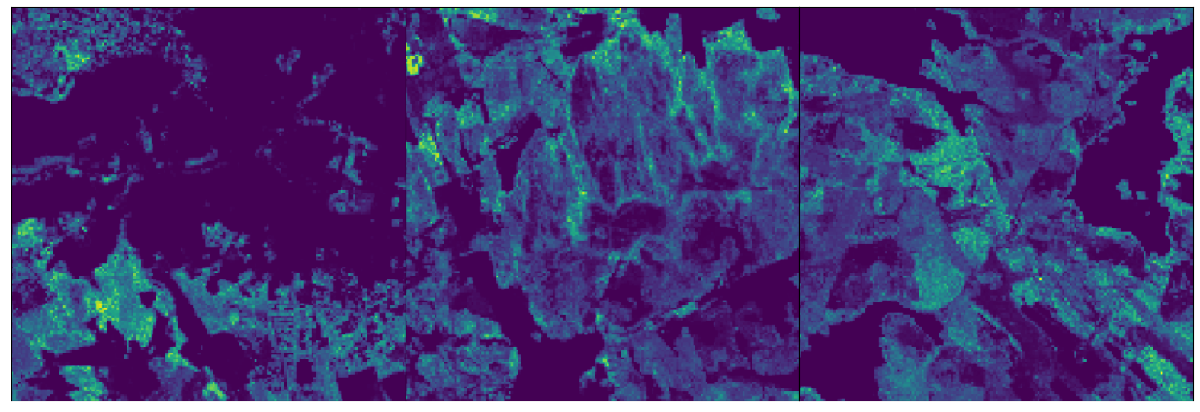[Fig 1.1: a visualization of AGBM in color. [Image derived from data provided by the Finnish Forest Centre under the CC BY 4.0 license]]
If you want to access and run this code, you can use the ‘Run in your browser’ and ‘Download Live Script’ buttons in the bottom right corner of this page.

## The Data

Each chip_id represents one patch of land in a given year. For each chip, you are provided approximately 24 satellite images and 1 AGBM image.
The satellite imagery comes from two satellites called Sentinel-1 (S1) and Sentinel-2 (S2), covering nearly 13,000 patches of forest in Finland from 2017 to 2021. Each chip is 2,560 by 2,560 meters, and the images of these chips are 256 by 256 pixels, so each pixel represents a 10 by 10 meter area of land within the chip. You are provided a single image from each satellite for each calendar month. For S1, each image is generated by taking the mean across all images acquired by S1 for the chip during that time. For S2, you are provided the best image for each month.
The AGBM image serves as the label for each chip in a given year. Just like the satellite data, the AGBM data is provided in the form of images that cover 2,560 meter by 2,560 meter areas at 10 meter resolution, which means they are 256 by 256 pixels in size. Each pixel in the satellite imagery corresponds to a pixel in the AGBM image with the same chip ID.
For the competition, you will use this data to train a model that can predict this AGBM value when provided only the satellite imagery. To learn more about the images, features, labels and submission metrics, head over to the challenge’s Problem Description page!

### Preview the Data

To understand the data that we will be working with, let’s look at a few example images for a specific chip_id. In the sections below, the images correspond to chip_id 0a8b6998.

First, define a variable that points to the S3 bucket so that we can access the data. You can find this path in the ‘biomassters_download_instructions.txt’ file provided on the data download page. Make sure this is the path for the entire bucket, not any specific folder – it should start with ‘s3://’. This will be used throughout the blog

% Example path, you will need to replace this
s3Path = ‘s3://competition/file/path/’;

#### Sentinel-1:

For each chip_id, we expect to see 12 images from Sentinel-1 with the naming convention {chip_id}_S1_{month}, where month is a value between 00 and 11. There are cases where there may be missing data, which could result in one or more of these images missing.
Each Sentinel-1 image has four bands, where each band is one 256×256 matrix that contains a specific measurement for the chip. Let’s visualize each band of one of these S1 images:
exampleS1Path = fullfile(s3Path, ‘train_features’, ‘0a8b6998_S1_00.tif’);
% To visualize each layer, rescale the values of each pixel to be between 0 and 1
% Darker pixels indicate lower values, ligher pixels indicate higher values
montage(rescale(exampleS1));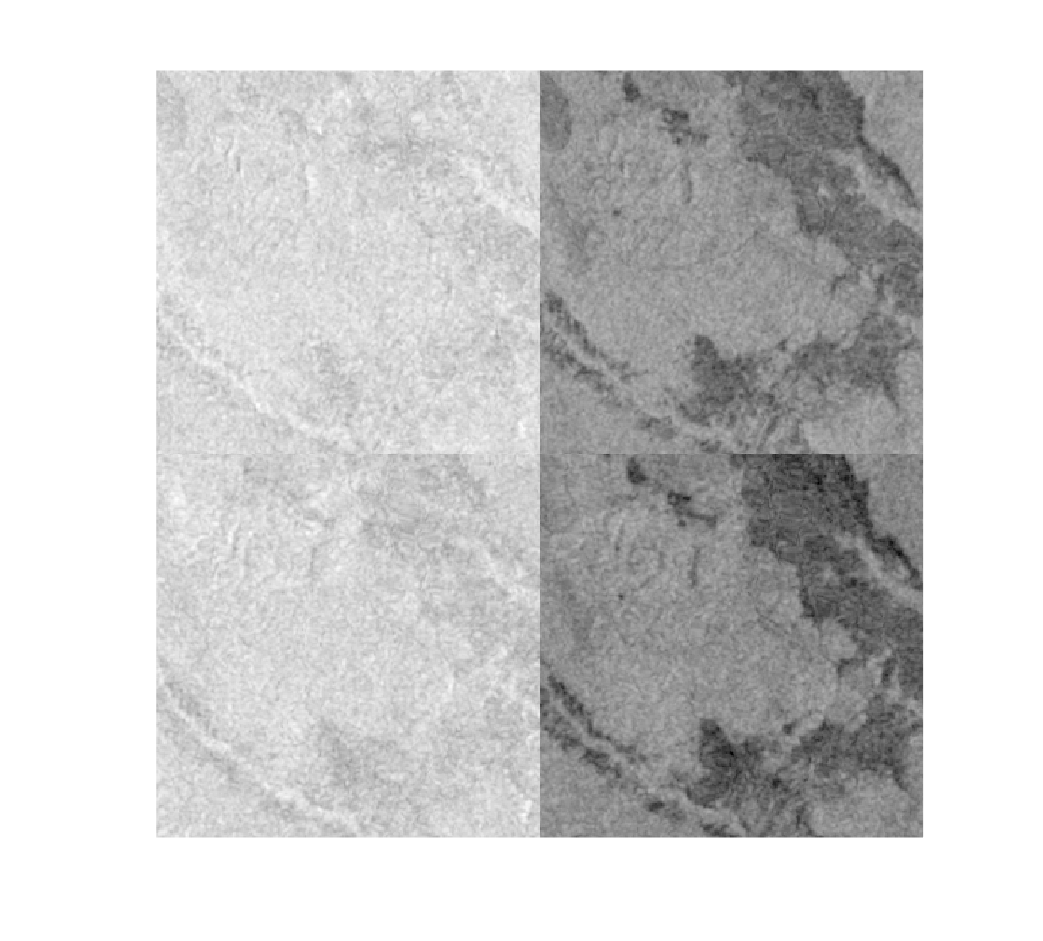#### Sentinel-2:

Much like Sentinel-1, for each chip_id, we expect to see 12 images from Sentinel-2 with the naming convention {chip_id}_S2_{month}, where month is a value between 00 and 11. There are cases where there may be missing data, which could result in one or more of these images missing.
Each Sentinel-2 image has 11 bands, where each band is one 256×256 matrix that contains a specific measurement for the chip. Let’s visualize each band of one of these S2 images:
exampleS2Path = fullfile(s3Path, ‘train_features’, ‘0a8b6998_S2_00.tif’);
% To visualize each layer, rescale the values of each pixel to be between 0 and 1
% Darker pixels indicate lower values, ligher pixels indicate higher values
montage(rescale(exampleS2));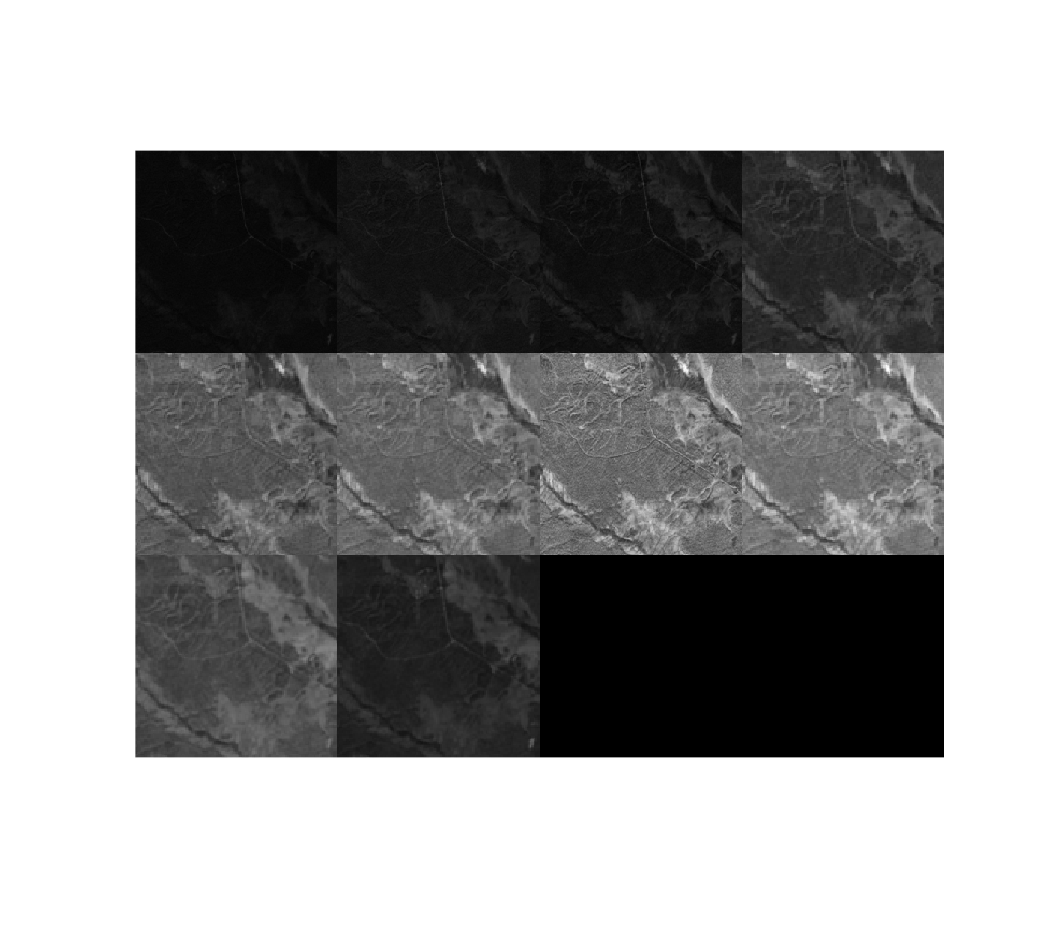#### AGBM:

For each chip_id, there will be one AGBM image, with the naming convention {chip_id)_agbm.tif. This image is a 256×256 matrix, where each element is a measurement of aboveground biomass in tonnes for that pixel. For 0a8b6998, it looks like this:
exampleAGBMPath = fullfile(s3Path, ‘train_agbm’, ‘0a8b6998_agbm.tif’);
% Since we only have to visualize one layer, we can use imshow
imshow(rescale(exampleAGBM))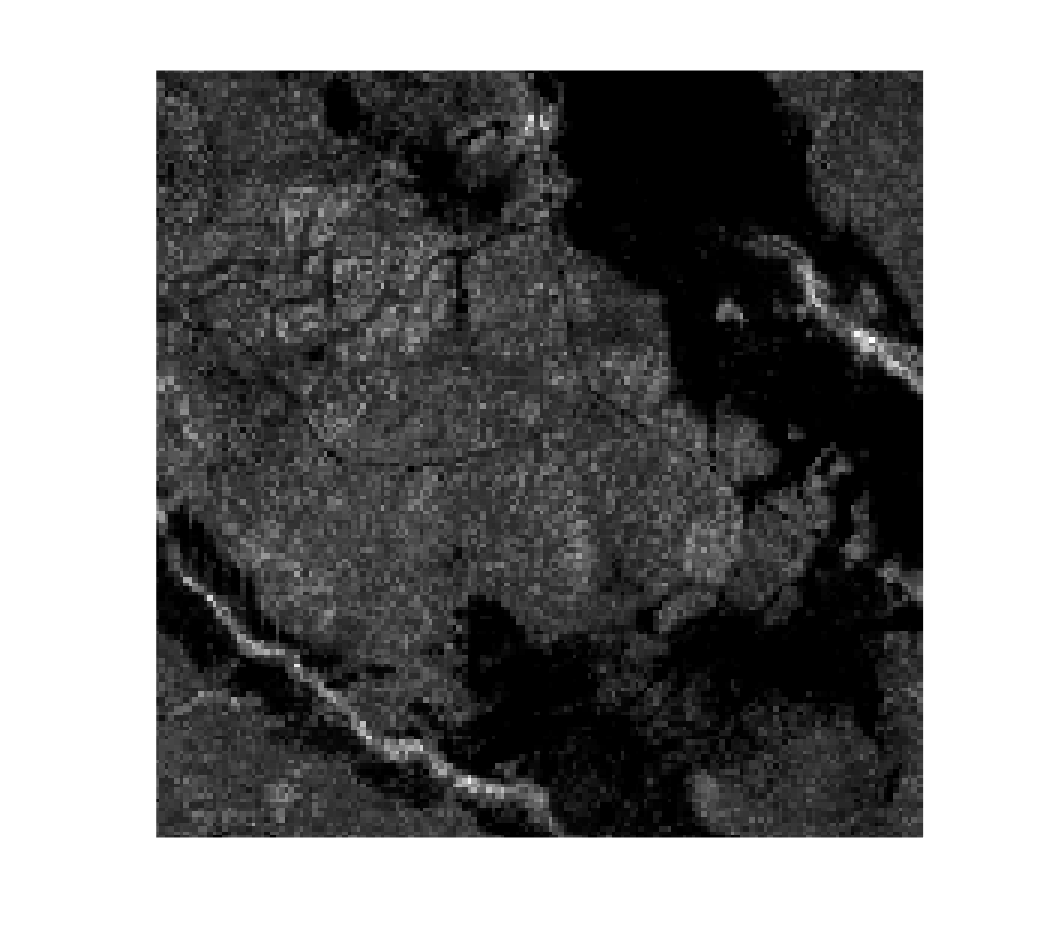## Import and Preprocess the Data

Before we can start building a model, we have to find a way to get the data into the MATLAB Workspace. The data for this competition is contained in a public Amazon S3™ bucket. The URL for this bucket will be provided once you have registered, so make sure you have signed up for the challenge so you can access the data. In total, all of the imagery provided takes up about 235GB of memory, which is too much to work with all at once. So that we can work with all of the data, I will be taking advantage of MATLAB’s imageDatastore, which allows us to read the data in one chip_id at a time and will make it easy to train a neural network later on. If you want to learn more about datastores, you can refer to the following resources:
We use the s3Path variable we created earlier to create a agbmFolder, which points specifically to the AGBM training data.
agbmFolder = fullfile(s3Path, ‘train_agbm’);
We can then use agbmFolder to create a datastore for our input (Satellite imagery) and output (AGBM imagery) data, named imInput and imOutput respectively. When you use an imageDatastore, you can change the way images from the specified directory are read in to the MATLAB Workspace using the ‘ReadFcn‘ option. Since I want to read one AGBM image but 24 satellite images at a time, I define a helper function readTrainingSatelliteData that reads the filename of the AGBM file we will read, which contains the chip_id, and instead reads in and preprocesses all corresponding satellite images. Then I use the built-in splitEachLabel function to divide the dataset into training, testing, and validation data, so that we can evaluate its performance during and after training. For this example, I chose to use 95% of the data for training, 2.5% for validation and 2.5% for testing because I wanted to use most of the data for training, but you can play around with these numbers.
The readTrainingSatelliteData helper function does the following:
1. Extracts the chip_id from the filename of the AGBM image that we will read
2. Reads in and orders all satellite images that correspond to this chip_id
3. Handles missing data. Since this is just our first model, I have decided to omit any images that contain missing data.
4. With the remaining data, finds the average value of each pixel for each band.
5. Rescales the values to be between 0 and 1. Each satellite has different units of measurement, which can make it difficult for some algorithms to learn from the data properly. By normalizing the data scale, it may allow the neural network to learn better.
This results in a single input image of size 256x256x15, where each 256×256 matrix represents the average values for one band from S1 or S2 over the course of the year. Since S1 has 4 bands and S2 has 11, this results in 15 matrices. This is a very simplified way to represent the data, as this will only be our starting model.
[inputTrain,inputVal,inputTest] = splitEachLabel(imInput,0.95,0.025);
For the output data, we will use the default read function, as we only need to read one image at a time and don’t need to do any preprocessing. Since we are passing the same directory to each datastore, we know that they will read the images in the same chip_id order. Once again, split the data into training, testing, and validation data.
imOutput = imageDatastore(agbmFolder, ‘LabelSource’, ‘foldernames’);
[outputTrain,outputVal,outputTest] = splitEachLabel(imOutput,0.95,0.025);
Once the data has been preprocessed, we combine the input and output sets so they may be used with our neural network later.
dsTrain = combine(inputTrain, outputTrain);
dsVal = combine(inputVal, outputVal);
dsTest = combine(inputTest, outputTest);
The preview function allows me to view the first item in the datastore, so that we can validate that the inputs (the first item) and outputs (the second item) are the sizes we are expecting:
sampleInputOutput = preview(dsTrain);
montage(rescale(sampleInputOutput{1})); % Input Data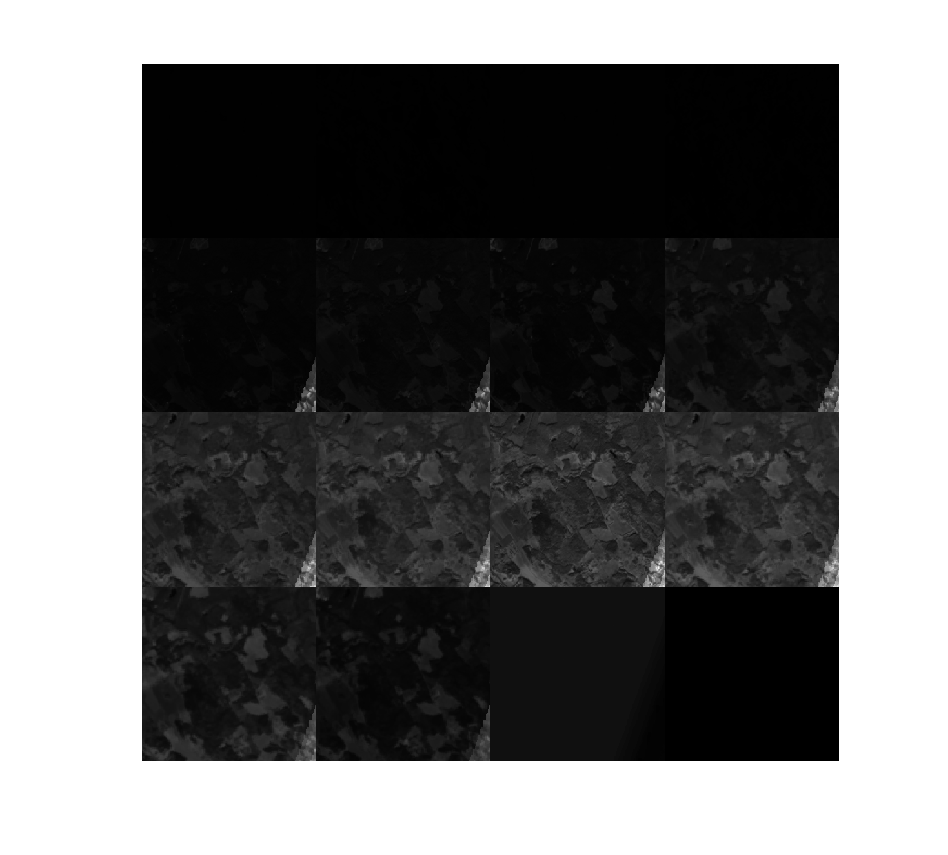imshow(rescale(sampleInputOutput{2})) % Output Data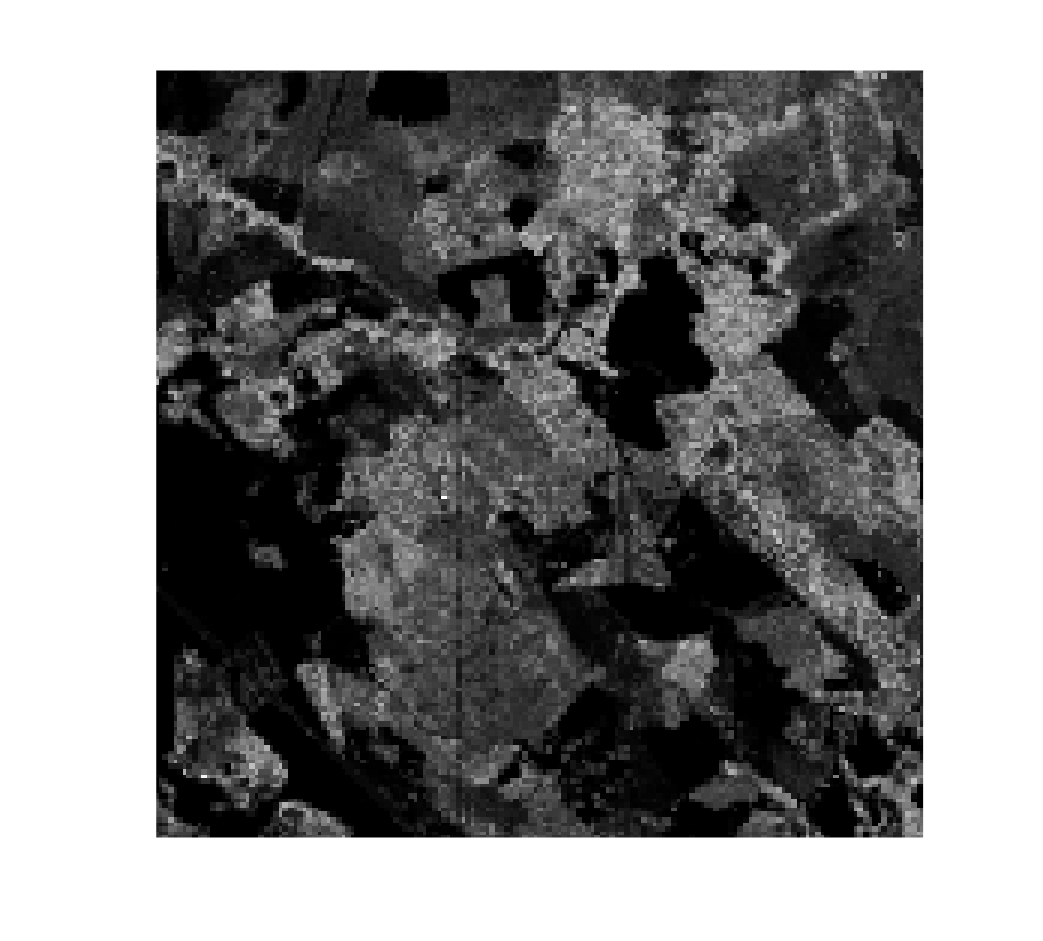## Create the Model

Now that the data is imported and cleaned up, we can get started on actually developing a neural network! This challenge is interesting, in that the inputs and outputs are images. Often, neural networks will be used to take an image as input and output a class (image classification) or maybe a specific value (image-to-one regression), as shown below: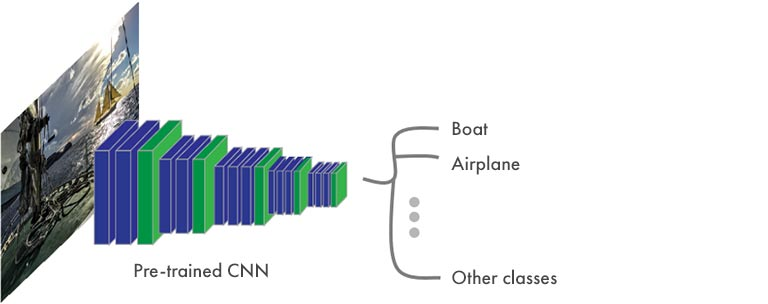[Fig 2.1: visualization of an image classification convolutional neural network]
In this challenge, we are tasked with outputting a new image, so our network structure will need to look a little different: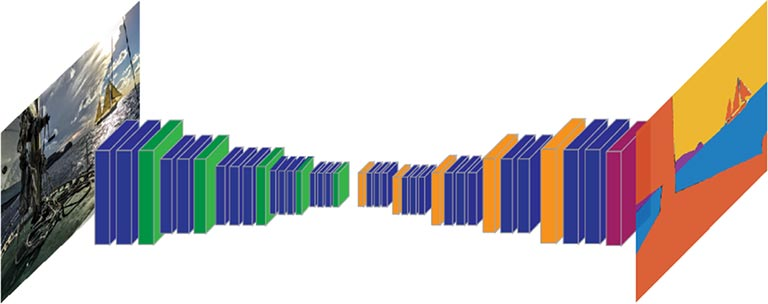[Fig 2.2: visualization of an image-to-image convolutional neural network]
No matter the type of network you’re using, there are two different ways you can make or edit a deep learning model: with the Deep Network Designer app or programmatically.

### Option 1: Create with the Deep Network Designer App

First, we have to choose a network architecture. For this blog, I have decided to create a starting network architecture using the ‘unetLayers‘ function. This function provides a network for semantic segmentation (an image-to-image classification problem), so it can be easily adapted for image-to-image regression. If you want to learn more about other starting architectures, check out this documentation page on Example Deep Learning Networks Architectures.
Since the input images will be 256x256x15, this must also be the input size of the network. For the other options, I chose an arbitrary number of classes since we will change the output layers anyway, and a starting depth of 3.
lgraph = unetLayers([256 256 15], 2,‘encoderDepth’,3);
From here, I can open the Deep Network Designer app and modify the model interactively. I like this option as it lets me visualize what the network looks like and it’s easier to see that I’ve made the changes I want.
deepNetworkDesigner(lgraph)
When the app opens, it should look similar to the image below. If it’s zoomed in on certain layers, you can zoom out to see the full network by pressing the space bar.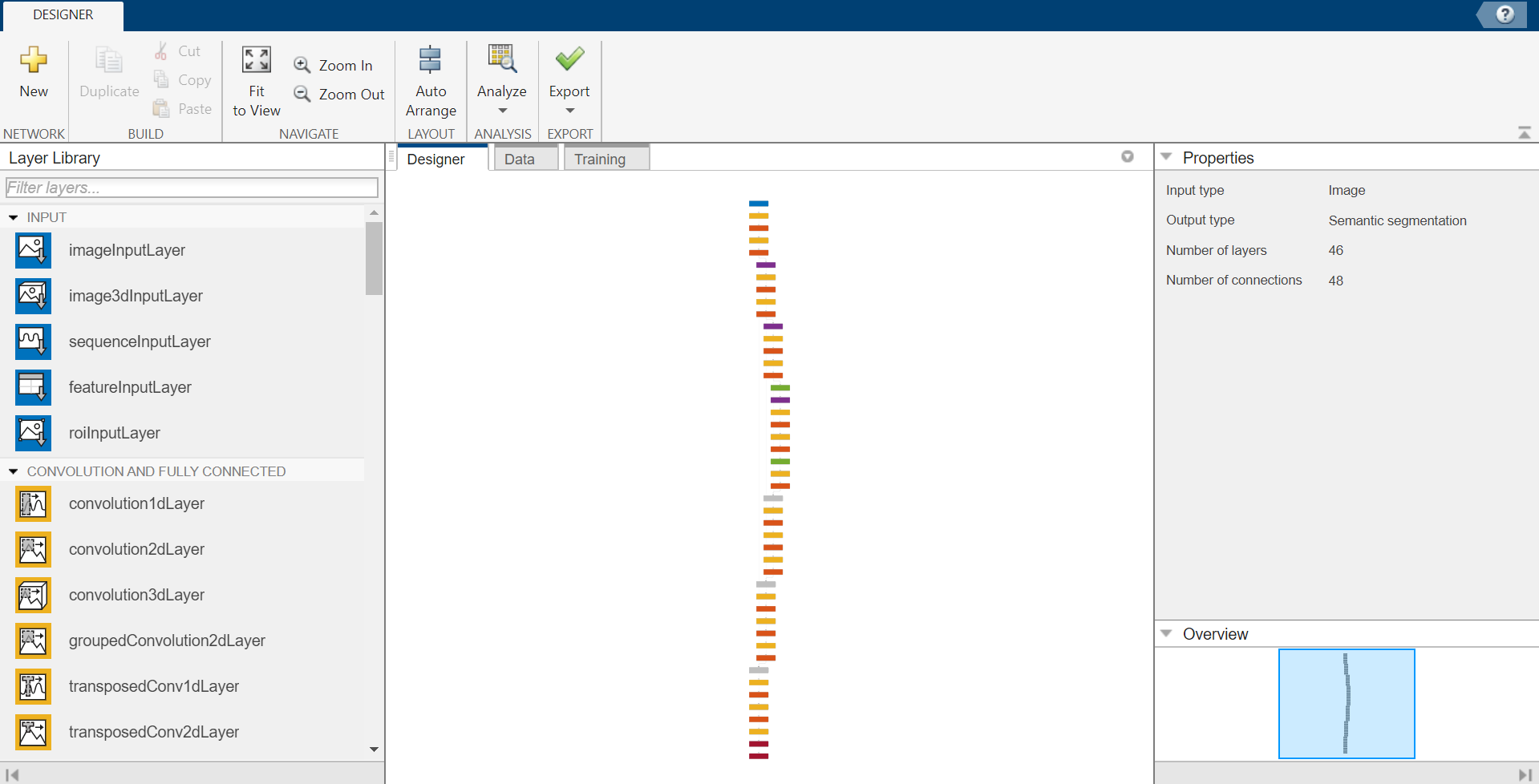[Fig 3.1: Deep Network Designer]
From here, remove the last two layers, and change the “Final-ConvolutionLayer” so that NumFilters is equal to 1. Some tips for using the app for this step:
• To zoom in or out, hold CTRL and scroll up or down on the mouse
• To delete a layer, click on it and hit the Backspace button on your keyboard.
• To modify a property of a layer, click on the layer. This will open a menu on the right that you can interact with.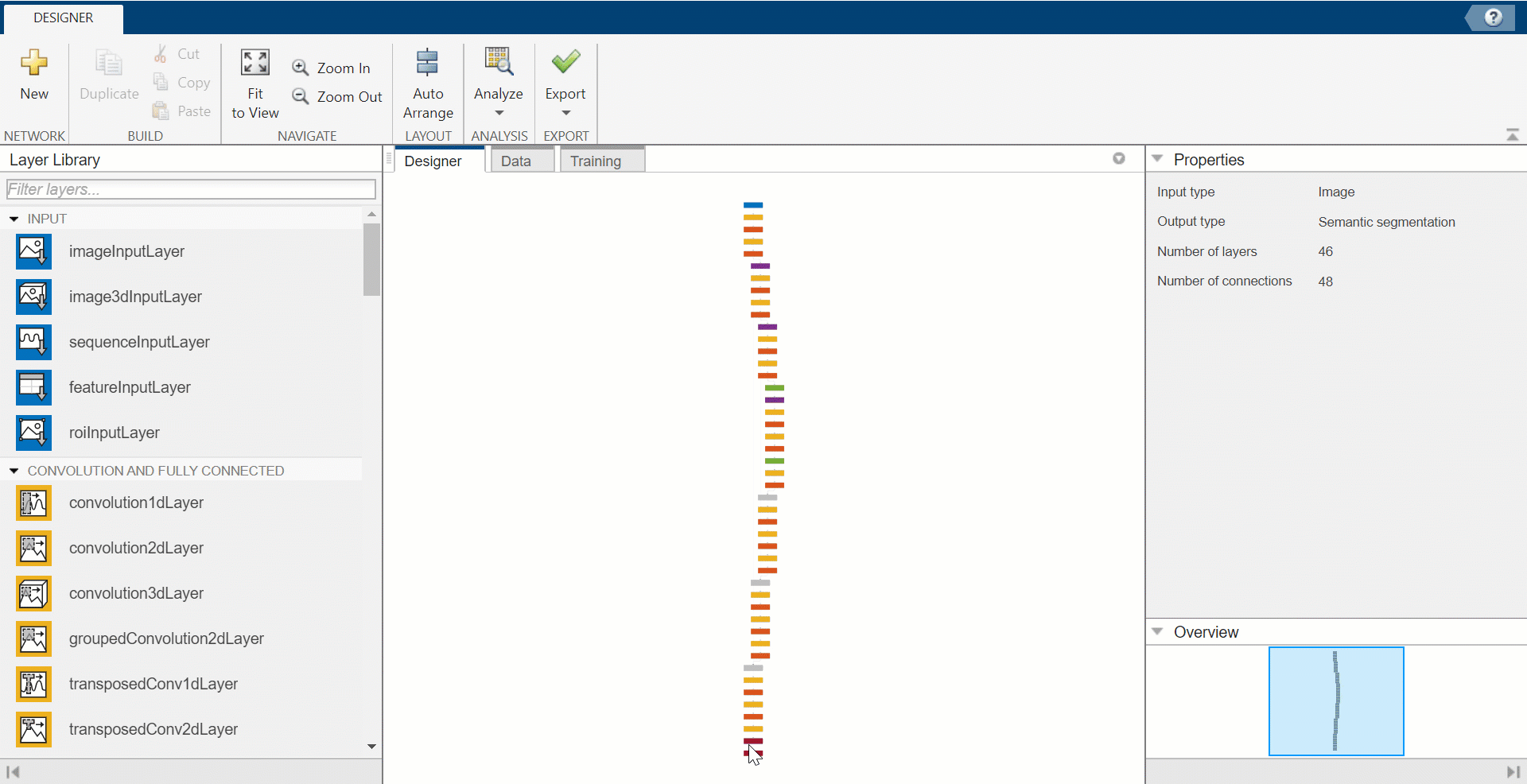[Fig 3.2: Removing and Modifying layers in Deep Network Designer]
It’s time to add in the regression layer: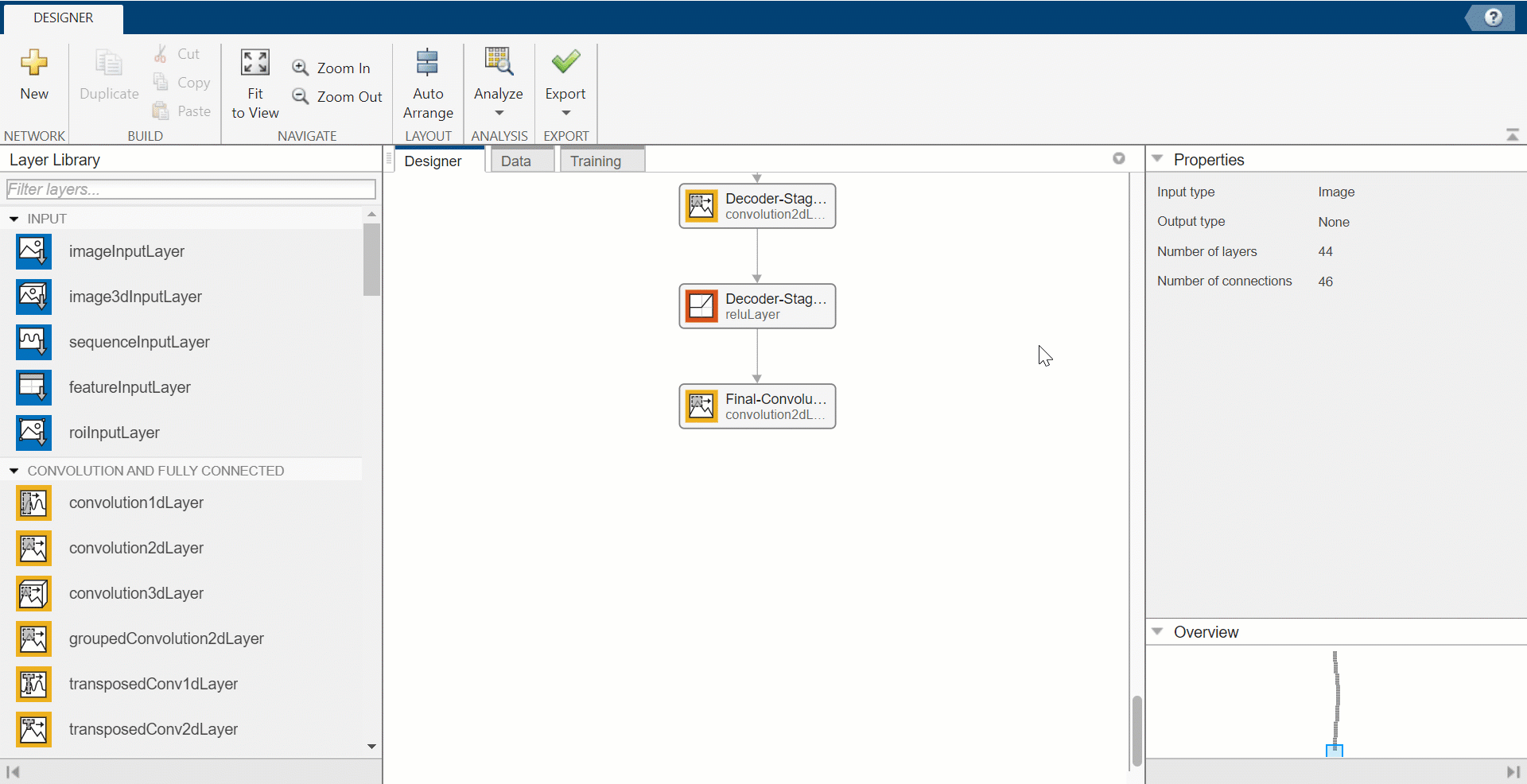[Fig 3.3: Adding a regression layer in Deep Network Designer]
Now, the model is done! It’s time to export it back into the MATLAB Workspace so it can be trained.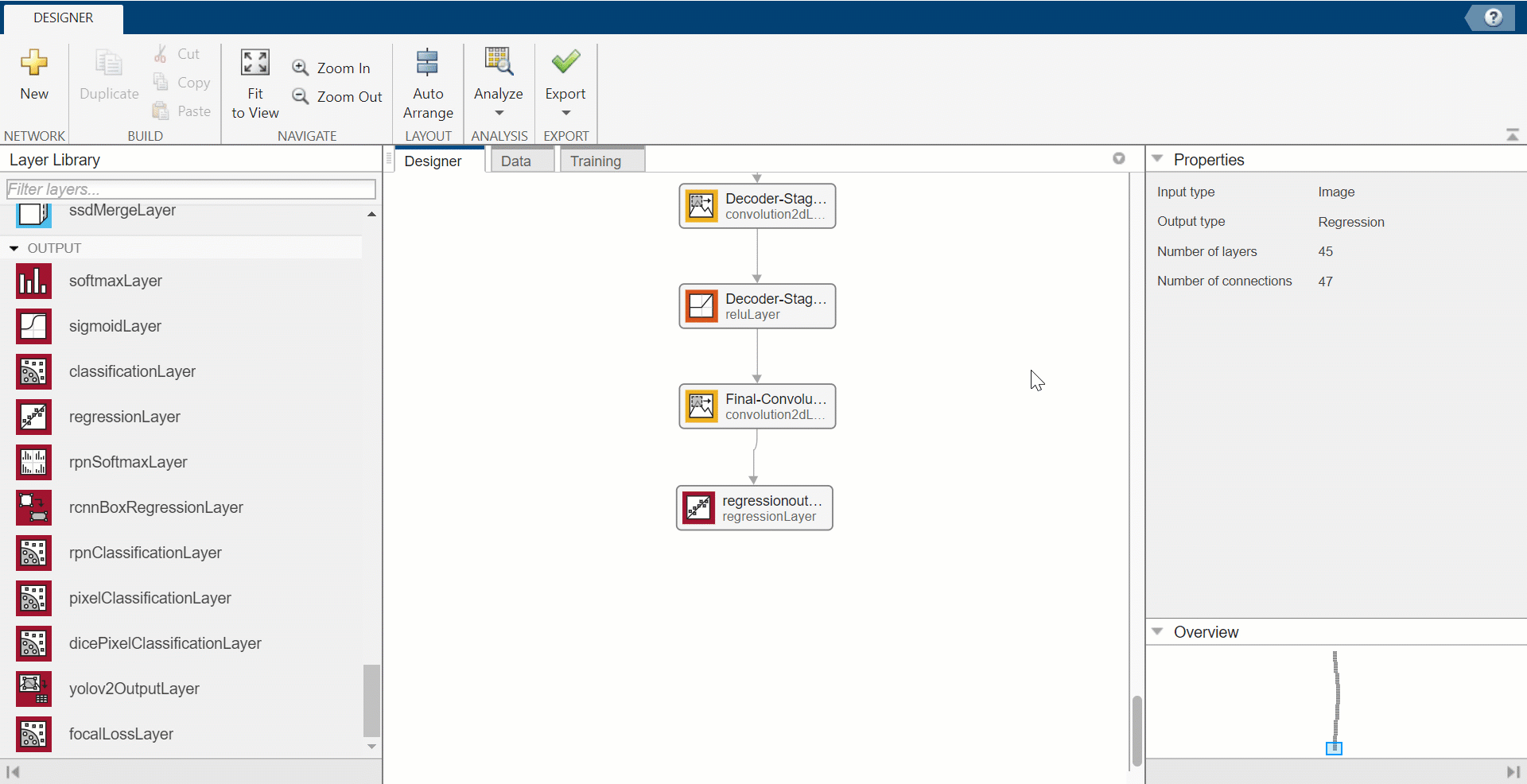[Fig 3.4: Exporting a model from Deep Network Designer]
Note: it will automatically be exported as lgraph_1.
If you want to get more creative with your model, this documentation page about Deep Network Designer has more details on how to use the app.

### Option 2: Create Programmatically

First, we have to choose a network architecture. For this blog, I have decided to create a starting network architecture using the ‘unetLayers‘ function. This function provides a network for semantic segmentation (an image-to-image classification problem), so it can be easily adapted for image-to-image regression. If you want to learn more about other starting architectures, check out this documentation page on Example Deep Learning Networks Architectures.
Since the input images will be 256x256x15, this must also be the input size of the network. For the other options, I chose an arbitrary number of classes since we will change the output layers anyway, and a starting depth of 3.
lgraph = unetLayers([256 256 15], 2,‘encoderDepth’,3);
Now we have to change the final few layers so that the model will perform regression instead of classification. I do this by removing the softmax and segmentation layers and replacing them with a new convolution layer and a regression layer. The new convolution layer has a single filter so that the final image output will be a single layer, and the regression layer tells MATLAB how to interpret the output and computes the model’s half-mean-squared-error. To learn more about converting classification networks into regression networks, you can refer to this resource: Convert Classification Network into Regression Network.
lgraph = lgraph.removeLayers(‘Softmax-Layer’);
lgraph = lgraph.removeLayers(‘Segmentation-Layer’);
finalConvolutionLayer = convolution2dLayer([1, 1], 1, ‘Name’, ‘Final-ConvolutionLayer-2D’);
lgraph = lgraph.replaceLayer(‘Final-ConvolutionLayer’, finalConvolutionLayer);
lgraph_1 = lgraph.connectLayers(‘Final-ConvolutionLayer-2D’,‘regressionLayer’);
Once the network is built, we can use the analyzeNetwork function to check for errors and visualize the network. This will open in a new window.
analyzeNetwork(lgraph_1);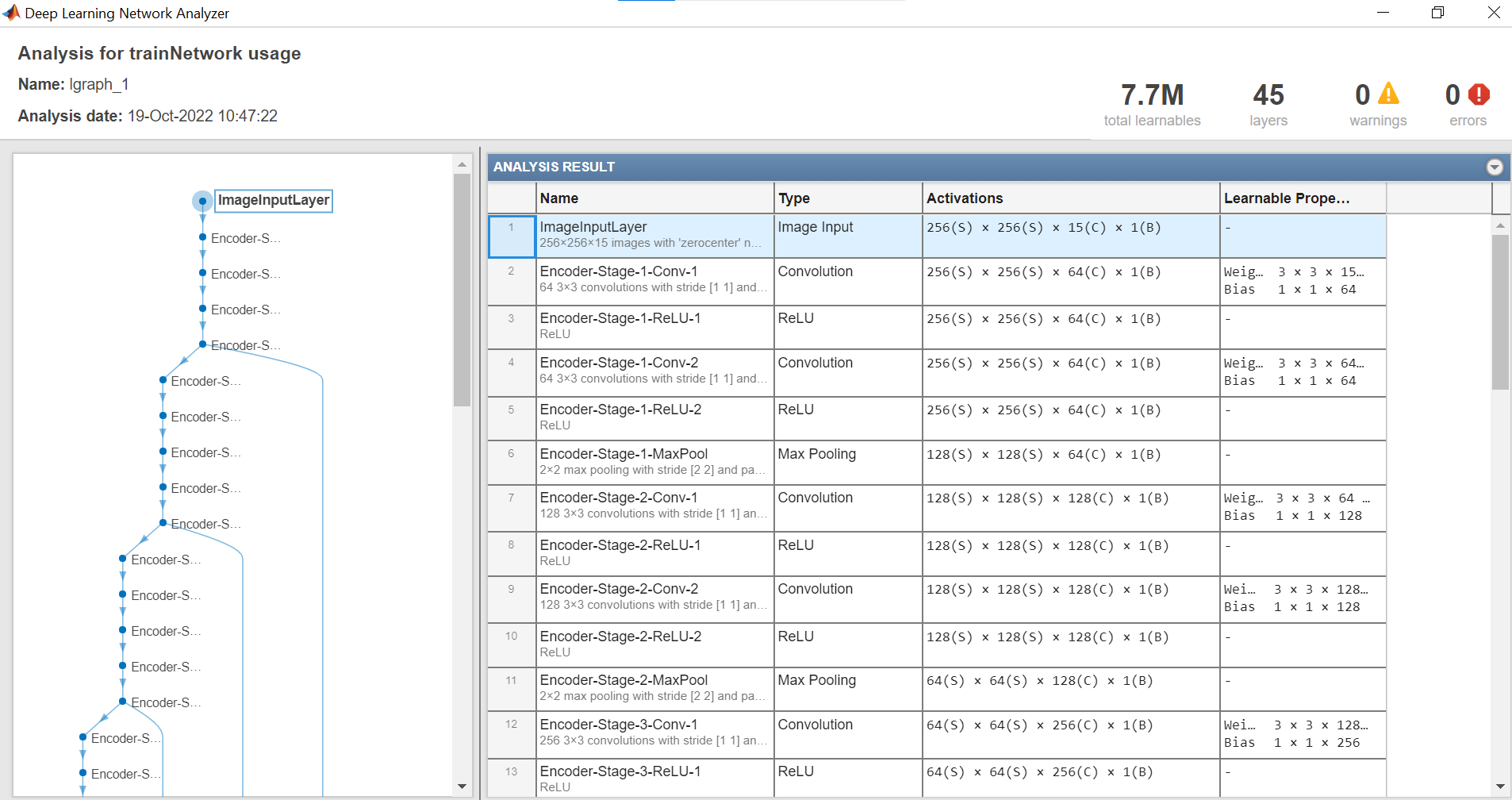[Fig 4: Analysis and visualization of lgraph_1]

## Set Training Preferences

Once all of the layers are sorted out, it’s time to set the training options. The trainingOptions function lets us specify which solver will train the model and how it will be trained, and it’s important to play around with these options when training a model. There are endless combinations you can choose from, but these are the ones that have worked best for me so far:
‘InitialLearnRate’, .0001,
‘MiniBatchSize’, 10,
‘MaxEpochs’, 50,
‘ValidationData’, dsVal,
‘OutputNetwork’, ‘best-validation-loss’,
‘Verbose’, false);
Note: if you want to see evaluation metrics and visualizations while the model is being trained, set ‘Verbose‘ to true and set ‘Plots’ to ‘training-progress’.
To learn more about what these training options do and how you can optimize them, you can refer this resource: Set Up Parameters and Train Convolutional Neural Network

## Train the Model

This step can be accomplished in only one line of code:
net = trainNetwork(dsTrain,lgraph_1,options)
While this is the shortest section of code, it will take several hours to train a deep learning model. If you have access to a supported GPU, I recommend using it – the ‘trainNetwork’ function will automatically utilize a supported GPU if one is detected. The following resource contains more information on GPUs and Deep Learning: Run MATLAB Functions on a GPU

## Evaluate the Model on New Data

Now we have a fully trained model that is ready to make predictions on the test data! Please note that the model I created was trained on only a subset of the training data, so the results you see in this section may look different than results you get if you run the same code.
To get output images from the test set, use the predict function.
ypred = predict(net,dsTest);
size(ypred)
ans = 1×4
256 256 1 24
The resulting ypred is a 4-D matrix. The first 3 dimensions represent each output image of size 256x256x1, and the last dimension represents how many of these images we have predicted. It is hard to tell how well our model performed just by looking at these numbers, so we can take a few extra steps to evaluate the network.

### Visualize

To access the first pair of satellite and AGBM images from the test set, use the preview function.
testBatch = preview(dsTest);
This will allow us to visualize a sample input image, the actual AGBM, and the associated predicted AGBM from the network to get a sense of how well the network is performing.
idx = 1;
predicted = ypred(:,:,:,idx);
ref = testBatch{idx,2};
montage({ref,predicted})
title(‘Expected vs Actual’);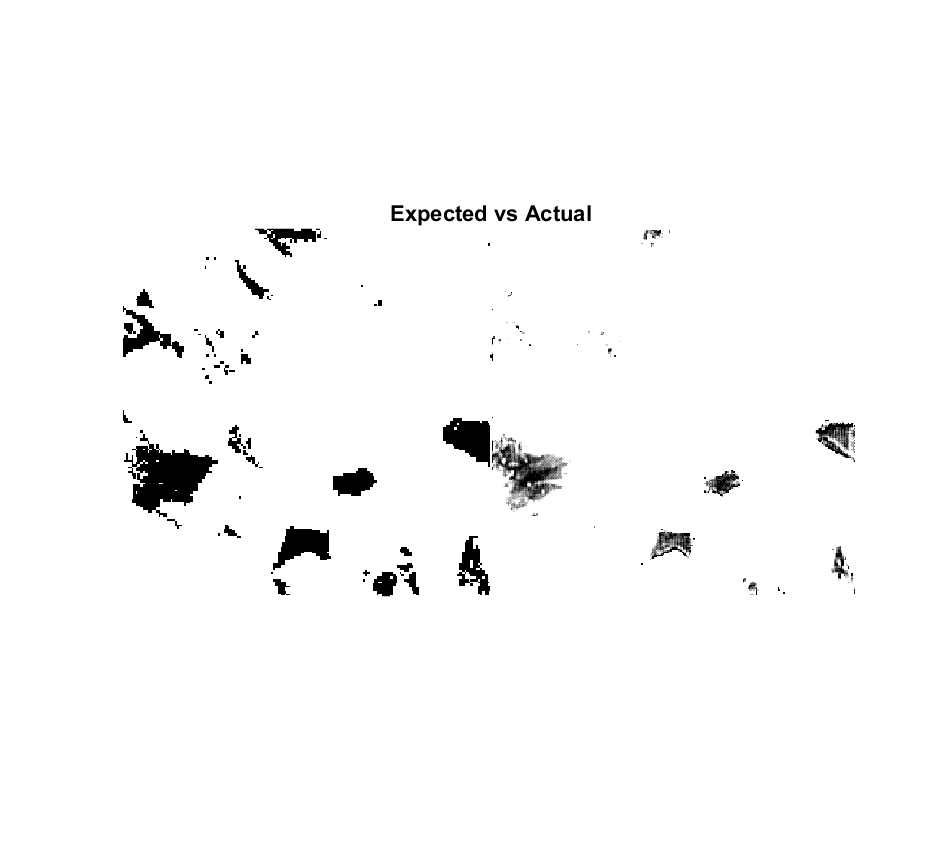While the images aren’t identical, we can definitely see similar shapes and shading! Since the output data is a measure of AGBM and not a representation of color, however, the values for each pixel aren’t between 0 and 1, so anything above 1 is being displayed as a white pixel. Let’s use the rescale function as we did before to get a better representation of the images so we can see more details and ensure that these higher values are still accurate.
rescaledPred = rescale(predicted);
rescaledRef = rescale(ref);
montage({rescaledRef,rescaledPred})
title(‘Expected vs Actual’);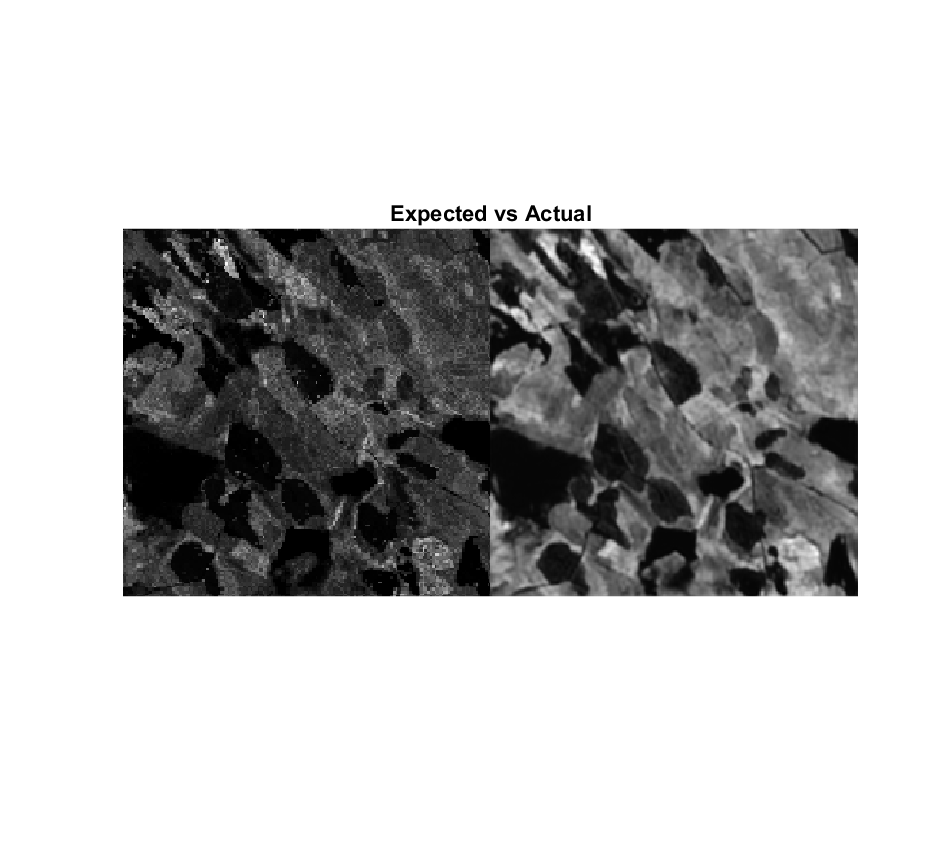Now that we can see much more detail, we can confirm that the network does a good job of matching the general shapes and countours of the expected output. We can also see, however, that the image produced by the network is generally brighter than the expected output, indicating that a lot of the values are higher than they should be.

### Calculate Performance Metrics

For the competition, your final score will be the average root-mean-square error (RMSE) of each image submitted. RMSE can be represented by the following formula:

– $E =$\sqrt{

E=1n∑i=1n|Ai-Fi|2

For a forecast array F and actual array A made up of n scalar observations.
Given this formula, we can calculate the RMSE for a given prediction with the following line of code:
rmse = sqrt(mean((ref(:) – predicted(:)).^2))
rmse = single
33.7451
The lower the RMSE, the better the model is. As you can see, there is still plenty of room for improvement of this model.

## Possible Next Steps for Improvement

Keep in mind that this network may not be the best because my main goal with this blog was to show how to use the imageDatastore and how to set up a network. But I do have a network that really tries, and there are lots of ways to keep trying things out and improving this network:
• Create a model that accepts more information! Right now we lose a lot of information from the raw training data, so finding a way to use more of it could result in a more informed model.
• Instead of ignoring data, find ways to fill it in. Do you make a copy of previous satellite images when one is missing? Fill it in with an average? There are lots of ways to approach this.
• Incorporate the cloud cover layer.
• Try out different model structures! One other example structure can be found here.
• Experiment with training options.
• Try different distributions of training, testing, and validation data.

## Predict on Test Data & Export Results

Once you have a model that you are happy with, you will need to use it to make predictions on the test data. To do this, we’ll need to first import and preprocess the data as we did above, then use the predict function to make predictions. Since we don’t have an ‘agbm’ folder to use as reference this time, the way we preprocess the data will have to look a little different.
To start, we will use the ‘features_metadata’ file provided to get a list of all test chip_ids.
testChips = testFeatures.chip_id;
[~, uniqueIdx, ~] = unique(testChips);
uniqueTestChips = testChips(uniqueIdx, :);
Then I make a new folder that will hold all of the predictions and a variable that points to this folder:
if ~exist(‘test_agbm’, ‘dir’)
mkdir test_agbm
end
% Include full file path, this is a placeholder – this should NOT be on the S3 bucket
outputFolder = ‘C:\DrivenData\..’;
Then, iterate through each chip_id and format the input data to match the expected format of our network (256x256x15), make predictions on the input data, then export each prediction as a TIFF file using the Tiff and write functions. For the competition, the expected names of these TIFF files is ‘{chip_id}_agbm.tif’.
for chipIDNum = 1:length(uniqueTestChips)
chip_id = uniqueTestChips{chipIDNum};
% Format inputs
% Make predictions
pred = predict(net, inputImage);
% Set up TIF file and export prediction
filename = [outputFolder, chip_id, ‘_agbm.tif’];
t = Tiff(filename, ‘w’);
% Need to set tag info of Tiff file
tagstruct.ImageLength = 256;
tagstruct.ImageWidth = 256;
tagstruct.Photometric = Tiff.Photometric.MinIsBlack;
tagstruct.BitsPerSample = 32;
tagstruct.SamplesPerPixel = 1;
tagstruct.SampleFormat = Tiff.SampleFormat.IEEEFP;
tagstruct.PlanarConfiguration = Tiff.PlanarConfiguration.Chunky;
tagstruct.Compression = Tiff.Compression.None;
tagstruct.Software = ‘MATLAB’;
setTag(t,tagstruct)
write(t, pred);
close(t);
end
And just like that, you’ve exported your predictions! To create a TAR file of these predictions, we can simply use the built-in tar function.
tar(‘test_agbm.tar’, ‘test_agbm’);
The resulting ‘test_agbm.tar’ is what you will submit for the challenge.
Thank you for following along with this starter code! We are excited to see how you will build upon it and create models that are uniquely yours. Feel free to reach out to us in the DrivenData forum or email us at studentcompetitions@mathworks.com if you have any further questions. Good luck!

## Helper Functions

outputFilenameParts = split(outputFilename, [“_”, “\”]);
chip_id = outputFilenameParts{end-1};
inputDir = fullfile(s3Path, ‘train_features\’);
correspondingFiles = dir([inputDir, chip_id, ‘*.tif’]);
% The satellite images range from 00-11, so preallocate a cell arrray
s1Data = cell(1, 12);
s2Data = cell(1, 12);
% Compile and order all data
for fileIdx = 1:length(correspondingFiles)
filename = correspondingFiles(fileIdx).name;
filenameParts = split(filename, [“_”, “\”, “.”]);
satellite = filenameParts{end-2};
fullfilename = strcat(inputDir, filename);
% Plus one because matlab starts at 1
idx = str2double(filenameParts{end-1}) + 1;
% Add all input images to ordered cell array
if satellite == ‘S1’
s1Data{idx} = im;
elseif satellite == ‘S2’
s2Data{idx} = im;
end
end
% Ignore missing data
for imgNum = 1:12
% Not enough bands
if size(s1Data{imgNum}, 3) ~= 4
s1Data{imgNum} = [];
elseif size(s2Data{imgNum}, 3) ~= 11
s2Data{imgNum} = [];
end
% Value of -9999
if ismember(-9999, s1Data{imgNum})
s1Data{imgNum} = [];
elseif ismember(-9999, s2Data{imgNum})
s2Data{imgNum} = [];
end
end
% Calculate average S1 data
totalImS1 = zeros(256, 256, 4);
for imgNum1 = 1:length(s1Data)
currIm = s1Data{imgNum1};
if ~(isempty(currIm))
totalImS1 = totalImS1 + currIm;
end
end
avgImS1 = totalImS1 ./ length(s1Data);
% Calculate average S2 data
totalImS2 = zeros(256, 256, 11);
for imgNum2 = 1:length(s2Data)
currIm = s2Data{imgNum2};
if ~(isempty(currIm))
totalImS2 = totalImS2 + currIm;
end
end
avgImS2 = totalImS2 ./ length(s2Data);
% Combine all bands into one 15 band image
avgImS1S2 = cat(3, avgImS1, avgImS2);
% Rescale so the values are between 0 and 1
avgImS1S2 = rescale(avgImS1S2);
end
% Update to point to s3
inputDir = fullfile(s3Path, ‘test_features’);
correspondingFiles = dir([inputDir, chip_id, ‘*.tif’]);
% The satellite images range from 00-11, so preallocate a cell arrray
s1Data = cell(1, 12);
s2Data = cell(1, 12);
% Compile and order all data
for fileIdx = 1:length(correspondingFiles)
filename = correspondingFiles(fileIdx).name;
filenameParts = split(filename, [“_”, “\”, “.”]);
satellite = filenameParts{end-2};
fullfilename = strcat(inputDir, filename);
% Plus one because matlab starts at 1
idx = str2double(filenameParts{end-1}) + 1;
% Add all input images to ordered cell array
if satellite == ‘S1’
s1Data{idx} = im;
elseif satellite == ‘S2’
s2Data{idx} = im;
end
end
% Ignore missing data
for imgNum = 1:12
% Not enough bands
if size(s1Data{imgNum}, 3) ~= 4
s1Data{imgNum} = [];
elseif size(s2Data{imgNum}, 3) ~= 11
s2Data{imgNum} = [];
end
% Value of -9999
if ismember(-9999, s1Data{imgNum})
s1Data{imgNum} = [];
elseif ismember(-9999, s2Data{imgNum})
s2Data{imgNum} = [];
end
end
% Calculate average S1 data
totalImS1 = zeros(256, 256, 4);
for imgNum1 = 1:length(s1Data)
currIm = s1Data{imgNum1};
if ~(isempty(currIm))
totalImS1 = totalImS1 + currIm;
end
end
avgImS1 = totalImS1 ./ length(s1Data);
% Calculate average S2 data
totalImS2 = zeros(256, 256, 11);
for imgNum2 = 1:length(s2Data)
currIm = s2Data{imgNum2};
if ~(isempty(currIm))
totalImS2 = totalImS2 + currIm;
end
end
avgImS2 = totalImS2 ./ length(s2Data);
% Combine all bands into one 15 band image
avgImS1S2 = cat(3, avgImS1, avgImS2);
% Rescale so the values are between 0 and 1
avgImS1S2 = rescale(avgImS1S2);
end

|